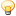A Flash Developer Resource Site

1. ##Percentage with sliders

Hello everyone, i need to make this work for Flash Player 6, so it has to be AS2.
i got this on the main scene, two dynamic textfields called ratio and ratio2
Now when i use a slider i want the ratio to go from 0-100 but at the same time i want the ratio2 to go from 100 and back to 0, so lets say i have it in the middle it should read 50 in ratio and 50 in ratio2

this is the code for the main scene:

this.onEnterFrame=function(){
ratio.text=mySlider.ratio;
ratio2.text=mySlider.ratio2;
}

And in the movieClip of the Slider i got this:

this.ratio=0 ;
this.ratio2=100;
dragger.onPress=function(){
this.startDrag(true,0,0,line._width,0);
this.onEnterFrame=function(){
ratio=Math.round(this._x);
ratio2=Math.round(this._x);
}
}
dragger.onRelease=dragger.onreleaseOutside=stopDra g;

it works well on ratio but as the code is now, i get same numbers in both ratio and ratio2, and i can't figure out how the code would be instead of the

ratio2=Math.round(this._x);

Can someone help me on this....THANKS a million.

Regards
Jimmy
aka MirrormanReply With Quote

2. ## i found the solution my self :-)

Ohh so i figured it out :-)

This works.

ratio2=ratio-100;Reply With Quote

math, percentage, ratio, slider, sliders####Posting Permissions

• You may not post new threads
• You may not post replies
• You may not post attachments
• You may not edit your posts
•

 » Home » Movies » Tutorials » Submissions » Board » Links » Reviews » Feedback » Gallery » Fonts » The Lounge » Sound Loops » Sound FX » About FK » Sitemap